2012-01-13

``````    var _alert = window.alert;
if(isDebug){
console.log(param);
}else{
}
}
``````

``````    _alert = window.alert;
``````

``````    var a = [1,2,3];
var b = a;
a.push(4);
console.log(a,b);//[1,2,3,4],[1,2,3,4]
``````

``````    var a = 1;
var b = a;
console.log(a,b);
``````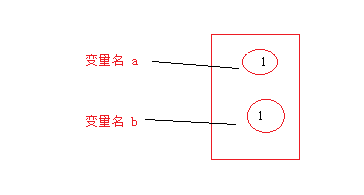``````    var a = 1;
var b = a;
a = 2;
console.log(a,b);
``````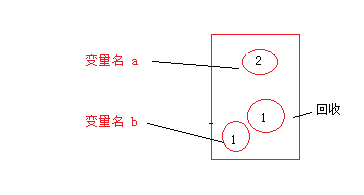``````    var a = [1,2,3];
var b = a;
console.log(a,b);
``````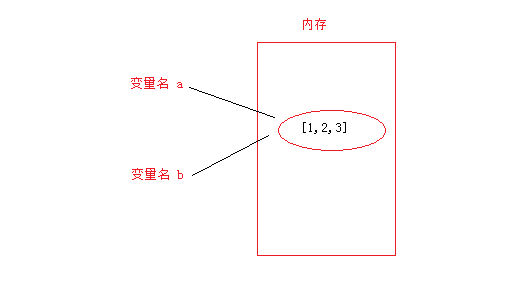继续：

``````    var a = [1,2,3];
var b = a;
a.push(4);
console.log(a,b);
````````````    var a = [1,2,3];
var b = a;
a = [1,2,3];
console.log(a,b);
``````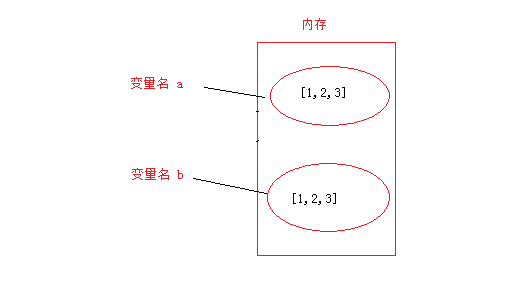``````    var a = [1,2,3];
var b = a;
a = [1,2,3];
a.push(4);
console.log(a,b);
``````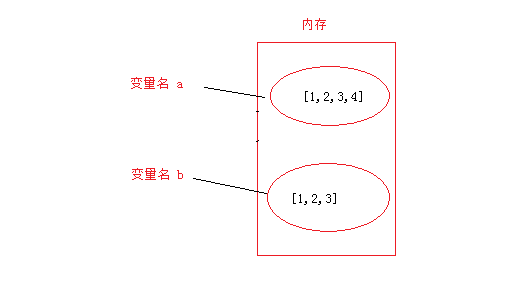``````    var _alert = window.alert;
if(isDebug){
console.log(param);
}else{
}
}
``````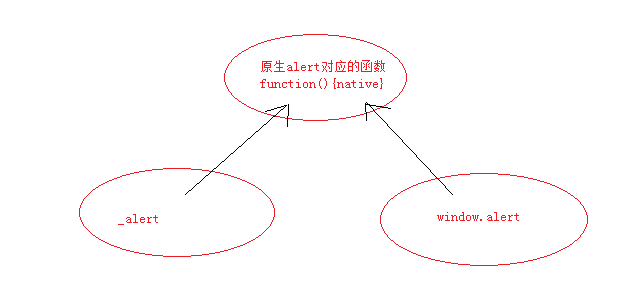``````    window.alert = function(param,isDebug){
//
}
``````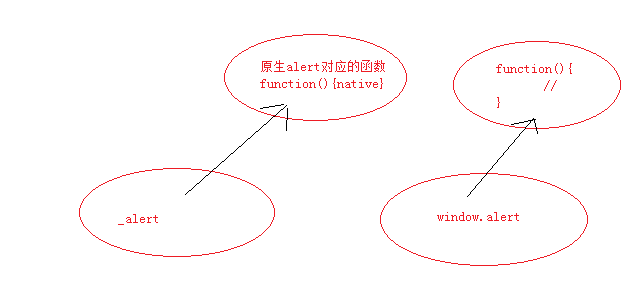``````    var _alert = window.alert;
``````